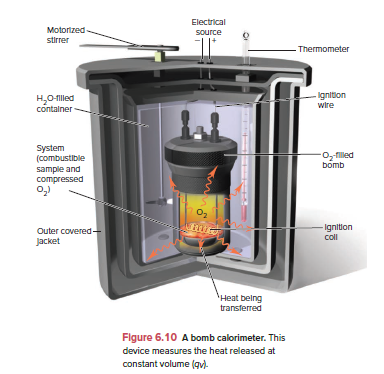# Problem: A chemical engineer burned 1.520 g of a hydrocarbon in the bomb of a calorimeter (see Figure 6.10). The water temperature rose from 20.00°C to 23.55°C. If the calorimeter had a heat capacity of 11.09 kJ/K, what was the heat released (qV) per gram of hydrocarbon?

###### FREE Expert Solution

We’re being asked to calculate the heat released (qV) per gram of hydrocarbon in a bomb calorimeter. This is given by the equation:

where

Ccal = heat capacity of the calorimeter

m = mass of water (in grams)

ΔT = change in temperature = final T – initial T.

81% (280 ratings)###### Problem Details

A chemical engineer burned 1.520 g of a hydrocarbon in the bomb of a calorimeter (see Figure 6.10). The water temperature rose from 20.00°C to 23.55°C. If the calorimeter had a heat capacity of 11.09 kJ/K, what was the heat released (qV) per gram of hydrocarbon?Frequently Asked Questions

What scientific concept do you need to know in order to solve this problem?

Our tutors have indicated that to solve this problem you will need to apply the Constant-Volume Calorimetry concept. You can view video lessons to learn Constant-Volume Calorimetry. Or if you need more Constant-Volume Calorimetry practice, you can also practice Constant-Volume Calorimetry practice problems.

What professor is this problem relevant for?

Based on our data, we think this problem is relevant for Professor Massari's class at UMN.

What textbook is this problem found in?

Our data indicates that this problem or a close variation was asked in Chemistry: The Molecular Nature of Matter and Change - Silberberg 8th Edition. You can also practice Chemistry: The Molecular Nature of Matter and Change - Silberberg 8th Edition practice problems.